Download Presentation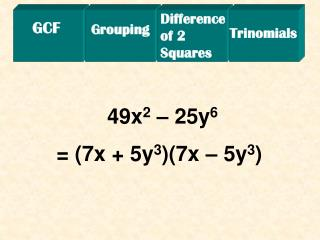GCF

# GCF - PowerPoint PPT Presentation

Difference of 2 Squares. GCF. Grouping. Trinomials. 49x 2 – 25y 6. = (7x + 5y 3 )(7x – 5y 3 ). Difference of 2 Squares. GCF. Grouping. Trinomials. 75x 4 + 25x 2 – 50x 5. = 25x 2 (3x 2 + 1 – 2x 3 ). Difference of 2 Squares. GCF. Grouping. Trinomials. x 2 – 15x + 56.I am the owner, or an agent authorized to act on behalf of the owner, of the copyrighted work described.
Download Presentation## GCF

An Image/Link below is provided (as is) to download presentation

Download Policy: Content on the Website is provided to you AS IS for your information and personal use and may not be sold / licensed / shared on other websites without getting consent from its author.While downloading, if for some reason you are not able to download a presentation, the publisher may have deleted the file from their server.

- - - - - - - - - - - - - - - - - - - - - - - - - - E N D - - - - - - - - - - - - - - - - - - - - - - - - - -
Presentation Transcript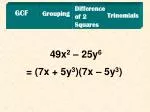Difference of 2 Squares

GCF

Grouping

Trinomials

49x2 – 25y6

= (7x + 5y3)(7x – 5y3)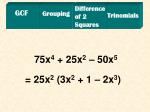Difference of 2 Squares

GCF

Grouping

Trinomials

75x4 + 25x2 – 50x5

= 25x2 (3x2 + 1 – 2x3)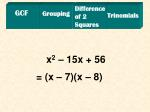Difference of 2 Squares

GCF

Grouping

Trinomials

x2 – 15x + 56

= (x – 7)(x – 8)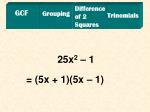Difference of 2 Squares

GCF

Grouping

Trinomials

25x2 – 1

= (5x + 1)(5x – 1)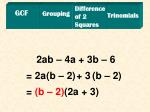Difference of 2 Squares

GCF

Grouping

Trinomials

2ab – 4a + 3b – 6

= 2a + 3

(b – 2) (b – 2)

= (b – 2)(2a + 3)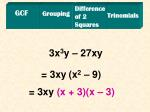Difference of 2 Squares

GCF

Grouping

Trinomials

3x3y – 27xy

= 3xy

(x2 – 9)

= 3xy (x + 3)(x – 3)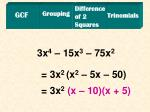Difference of 2 Squares

Grouping

GCF

Trinomials

3x4 – 15x3 – 75x2

= 3x2

(x2 – 5x – 50)

= 3x2(x – 10)(x + 5)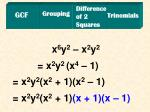Difference of 2 Squares

Grouping

GCF

Trinomials

x6y2 – x2y2

= x2y2

(x4 – 1)

= x2y2(x2 + 1)

(x2 – 1)

= x2y2(x2 + 1)(x + 1)(x – 1)